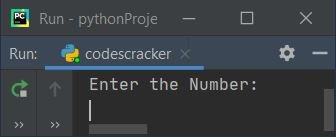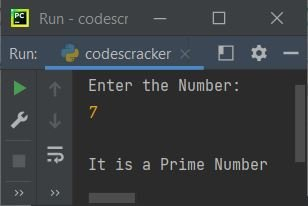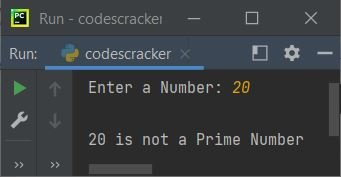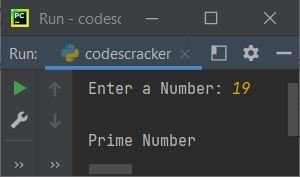# Python Program to Check Prime Number

In this article, we've created some programs in Python, to check whether a number entered by user is a Prime number or not. Here are the list of programs:

• Check Prime Number using for loop
• Using while loop
• Using Function
• Using Class

Note - Any natural number say x can be called as a Prime number, if it is greater than 1 and is not a product of two smaller natural numbers. For example, 2, 3, 5, 7, 11, 13 etc. are all prime numbers

## Check Prime Number using for Loop

To check whether a given number is a prime number or not in Python, you have to ask from user to enter the number, then check and print the message as shown in the program given below:

The question is, write a Python program to check prime number using for loop. Here is its answer:

```print("Enter the Number: ")
num = int(input())

p = 0
for i in range(2, num):
if num%i==0:
p = 1
break

if p==0:
print("\nIt is a Prime Number")
else:
print("\nIt is not a Prime Number")```

Here is its initial output:Now supply the input say 7 and press `ENTER` key to check whether it is a prime number or not. And print the message as shown in the snapshot given below:The range(2, num) states that the statements present in the body of for loop, gets executed num-2 number of times with value of i from 2 to one less than num's value.

#### Modified Version of Previous Program

This is the modified version of previous program. In this program, we've added two extra things. That are end and str(). The end is used to skip printing of an automatic newline. And str() is used to convert any type of value to a string type:

```print("Enter a Number: ", end="")
n = int(input())

p = 0
for i in range(2, n):
if n%i==0:
p = 1
break

if p==0:
print("\n" +str(n)+ " is a Prime Number")
else:
print("\n" +str(n)+ " is not a Prime Number")```

Here is its sample run with user input, 20 as number:## Check Prime Number using while Loop

This program uses while loop to do the same job as of previous program. Since while loop only contains condition-checking part, therefore the initialization part is written before the loop, and update part is included inside its body. Rest of the things are similar to the program, that uses for loop

```print("Enter a Number: ", end="")
n = int(input())

p = 0
i = 2
while i<n:
if n%i==0:
p = 1
break
i = i+1

if p==0:
print("\n" +str(n)+ " is a Prime Number")
else:
print("\n" +str(n)+ " is not a Prime Number")```

## Check Prime Number using Function

This program uses a user-defined function, checkPrime(). It receives a number as its argument and returns 1 if argument's value is a prime number. That is, if the argument's value is divisible by any number from 2 to one less than the number itself, function returns 1.

```def checkPrime(x):
for i in range(2, x):
if n%i==0:
return 1

print("Enter a Number: ", end="")
n = int(input())

p = checkPrime(n)
if p==1:
print("\n" +str(n)+ " is not a Prime Number")
else:
print("\n" +str(n)+ " is a Prime Number")```

This program produces similar output as of previous program.

## Check Prime Number using Class

This program is created using a class named CodesCracker, an object-oriented feature of Python.

```class CodesCracker:
def checkPrime(self, x):
for i in range(2, x):
if n%i==0:
return 1

print("Enter a Number: ", end="")
n = int(input())

obj = CodesCracker()
p = obj.checkPrime(n)
if p==1:
print("\nNot Prime Number")
else:
print("\nPrime Number")```

Here is its sample run with user input, 19:An object obj is created of class CodesCracker to access its member function named checkPrime() through dot (.) operator.

#### Same Program in Other Languages

Python Online Test

« Previous Program Next Program »